## Separate Air Chamber Equations

Written by Drenchenator
Last updated on 2008-05-20

## Notes

Ever wonder what gauge pressure is inside your water gun? A simple application of Boyle's Law provides the answer.

## Variables Used

• P - Pressure
• V - Volume
• A - Area of the pump shaft
• n - Number of pumps
• Pa - Atmospheric pressure (about 14.7 psia)
• Vc - Total volume of pressure chambers
• Vp - Amount of water in each stroke of the pump
• Vw - Volume of water in chamber
• Va - Volume of air in chamber

## Derivation

### Givens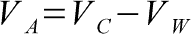The assumption is made that the entire chamber is filled with either water or air, comprising the entire volume of the chamber. The volume of air is then the difference between the volumes of the chamber and the water within it.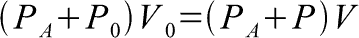The second assumption is made that the total number of molecules of air doesn't change even though they are compressed. This is where Boyle's Law comes in; the product of the absolute pressure and volume will remain the same if the temperature is held constant.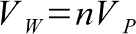The volume of water in the gun is the number of pumps time the volume of the pump. Simple enough to understand.

### Pumps to Pressure

Plug and chug from here on.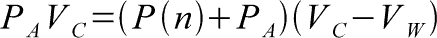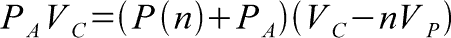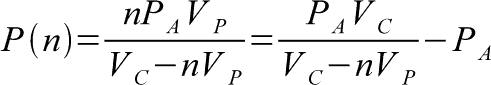### Pressure to Pumps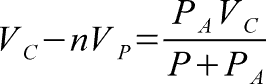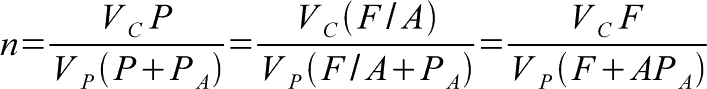### Absolute Maximum Number of Pumps

When the amount of water equals the total volume of the chamber, you can't pump anymore. It's impossible to achieve because the force and energy needed is infinite, so it tells you how many pumps you shouldn't have to do.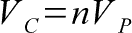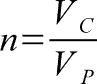### Average Force During a Pump

In an air pressure gun, the pressure increases while the pump you push the pump back. If you multiply The above pumps to pressure equation by the area of the pump as discussed in this article, you get the maximum force required to pump the gun for that pump. That is for the reason I listed above; the pressure increase during the pump and so the force increases. Since the equation is in increments of n for the number of pumps, it already assumed that it was a full pump.

The average above an interval is a simple use of the integral. I'm not going to explain how I got the answer--it was just a straightforward integral.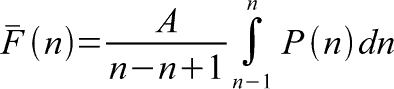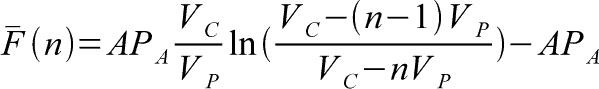### Work Done

As I've said before, the pressure increases with each pump. You may wonder how much work you are doing pumping your gun. Again, this is simple application of the integral and the pressure-volume work equation.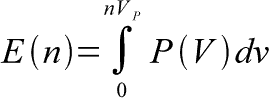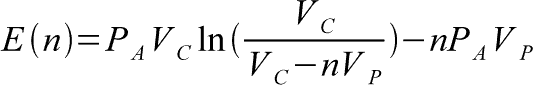## Equations

The gauge pressure in a separate air chamber water gun after n pumps:The number of pumps for a certain pressure or force:The absolute maximum number of pumps (never attainable):Average Pump ForceWork Done< http://www.sscentral.org/physics/separate_air.html >